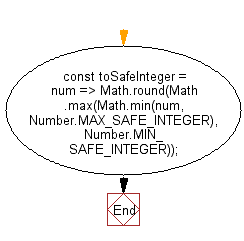# JavaScript: Convert a value to a safe integer

## JavaScript fundamental (ES6 Syntax): Exercise-121 with Solution

Write a JavaScript program to convert a value to a safe integer.

• Use Math.max() and Math.min() to find the closest safe value.
• Use Math.round() to convert to an integer.

Sample Solution:

JavaScript Code:

``````//#Source https://bit.ly/2neWfJ2
const toSafeInteger = num =>
Math.round(Math.max(Math.min(num, Number.MAX_SAFE_INTEGER), Number.MIN_SAFE_INTEGER));

console.log(toSafeInteger('3.2'));
console.log(toSafeInteger(Infinity));
```
```

Sample Output:

```3
9007199254740991
```

Pictorial Presentation:Flowchart:Live Demo:

See the Pen javascript-basic-exercise-121-1 by w3resource (@w3resource) on CodePen.

Improve this sample solution and post your code through Disqus

What is the difficulty level of this exercise?

Test your Programming skills with w3resource's quiz.

﻿

## JavaScript: Tips of the Day

Chunks an array into n smaller arrays

Example:

```const tips_chunkIntoN = (arr, n) => {
const size = Math.ceil(arr.length / n);
return Array.from({ length: n }, (v, i) =>
arr.slice(i * size, i * size + size)
);
}
console.log(tips_chunkIntoN([1, 2, 3, 4, 5, 6, 7,8], 4));
```

Output:

```[[1,2],[3,4],[5,6],[7,8]]
```